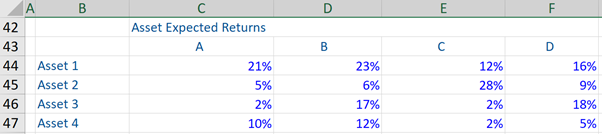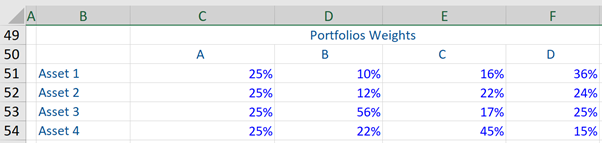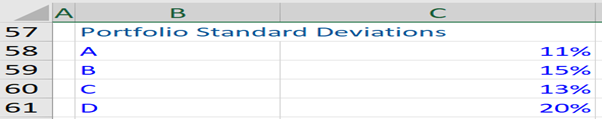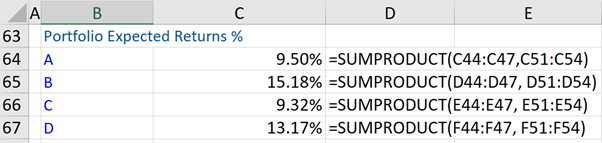## What is “Modern Portfolio Theory”?

Building a successful investment portfolio relies on balancing the risk and the reward within the investments. The Modern Portfolio Theory (MPT), pioneered by Harry Markowitz in 1952, is a theory that gives a framework on how to evaluate and analyze these two factors – which are the dominant drivers in investment decision-making. The investment theory’s underlying aim is to maximize expected return by selecting the weights of various assets or asset classes, and uses the only risk and return in its analysis. Many new theories have built upon the MPT and in today’s investment world, this theory remains very relevant.

Within the MPT framework of analysis, which is strictly based on risk versus return, an optimum portfolio will achieve the best possible risk-return tradeoff. Furthermore, different types of investors will inevitably position themselves differently along the risk return trade-off – depending primarily on their risk appetite.

## Key Learning Points

• Modern Portfolio Theory assumes that all investors are rational
• Investors can vary the portfolio weighting in various assets or asset classes, in order to determine optimal portfolios
• MPT analysis results in what is termed the “Efficient Frontier”
• The MPT is considered too simplistic by many and further theories have been built upon it

## Modern Portfolio Theory – Salient Features

The MPT assumes that all investors are rational i.e. given an acceptable level of risk, all investors attempt to achieve the highest level of return for that level of risk. Additionally, investors who are wanting a particular targeted level of return should seek out the lowest level of risk for that return level. This can be done by Mean-Variance Optimization (MVO).

The MVO is the process by which investors will allocate portfolios based on the risk-reward trade-off. This is done by investors varying the weightings in various assets or asset classes to determine which portfolios provide the best risk-return trade-off. Such analysis results in what is termed as the “Efficient Frontier”.

The Efficient Frontier is created graphically – if all portfolios of varying risks are plotted on a graph against the expected return for those portfolios. The top edge of the graph would represent the most efficient portfolio.

Modern Portfolio Theory – the Efficient Frontier. This is the set of efficient or optimal portfolios that an investor can choose from. Such portfolios offer the highest expected return for a defined level of standard deviation (risk), or the lowest standard deviation for a given level of expected return. Portfolios that lie below this frontier are not efficient or optimal, as they do not provide adequate returns commensurate to the level of risk.

Suppose we chart four portfolios A, B, C, and D against their respective expected return (Y-axis) and risk (i.e. standard deviation, X-axis). Now, if portfolio A and C have the same level of risk (i.e. standard deviation) but portfolio A produces a lower expected return, then this portfolio is inferior to portfolio C. Furthermore if we compare portfolios B and D, and both have the same expected return but portfolio B has a higher standard deviation (risk), then portfolio D is superior to portfolio B i.e. portfolio D is more optimal and efficient.

When we chart the outermost portfolios, we would plot the efficient frontier. Every investor in theory should only seek portfolios on the efficient frontier line.

No investor would likely choose to invest in a portfolio that is to the right of the efficient frontier as the portfolio on the frontier can offer the same return for a lower level of risk. Finally, where exactly would an investor choose to allocate on the efficient frontier, is determined by each investor’s risk tolerance and return objectives?

Having stated the above, the MPT is considered too simplistic by many, as it considers only two factors – risk and returns in analysis, rather than multiple factors. Further, the MPT is typically backward-looking.

## Modern Portfolio Theory – Example

Given below are portfolios A, B, C, and D. Each portfolio is made up of four assets. The individual expected return on these assets, along with their respective weightings in each of the four portfolios (A, B, C, and D), is also stated. Further, the standard deviation (risk) of each portfolio is given. Thereafter, the expected return on portfolios A, B, C, and D has been calculated below.After calculating the expected return on each portfolio (A, B, C, and D) and taking into account their respective standard deviation, we can infer that both portfolios C and D are inefficient. This is because other portfolios can attain a higher expected rate of return with lower risk.